# iCAMPResearchPaper_ObjectRecognition (2)

25 de Jul de 2016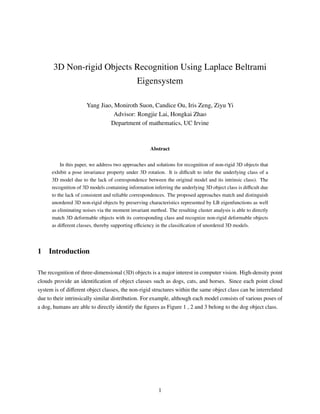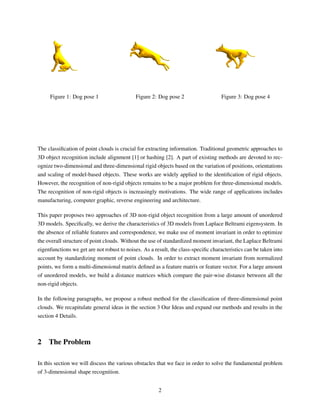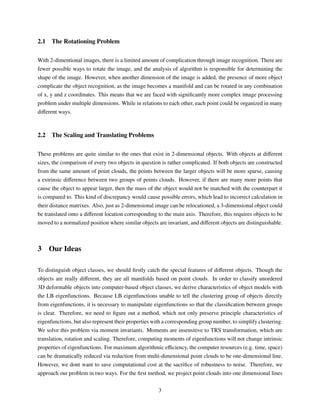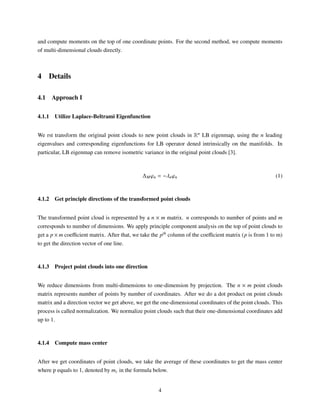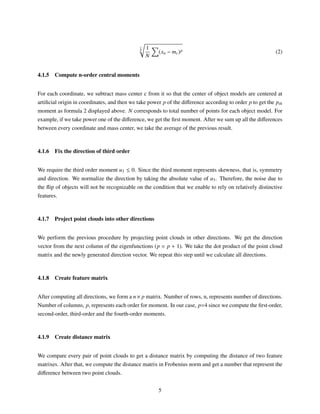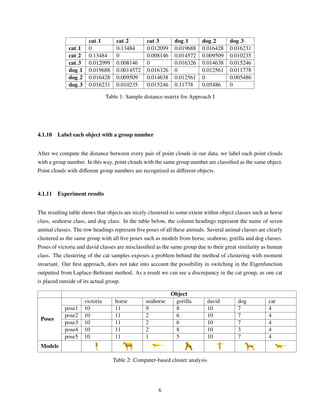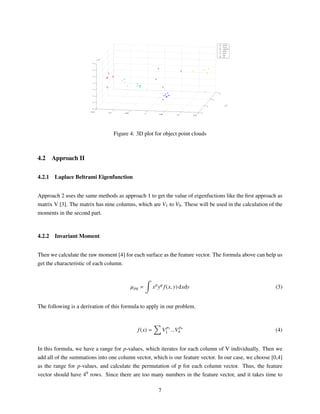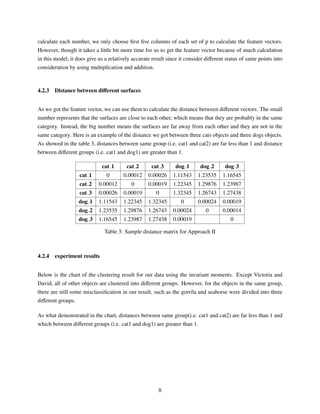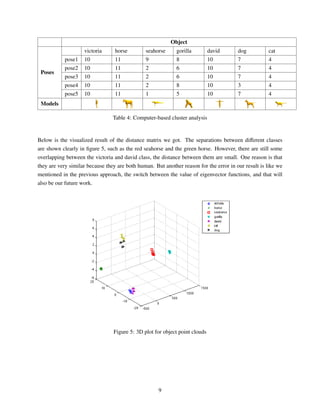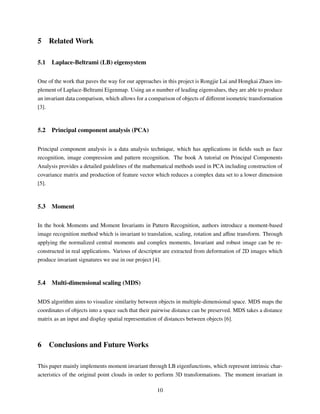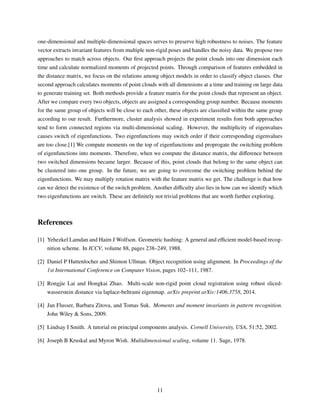Próximo SlideShare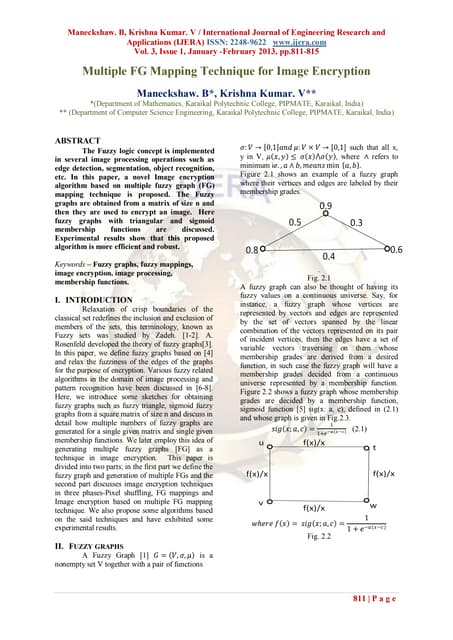Dt31811815
Cargando en ... 3
1 de 11

### iCAMPResearchPaper_ObjectRecognition (2)

1. 3D Non-rigid Objects Recognition Using Laplace Beltrami Eigensystem Yang Jiao, Moniroth Suon, Candice Ou, Iris Zeng, Ziyu Yi Advisor: Rongjie Lai, Hongkai Zhao Department of mathematics, UC Irvine Abstract In this paper, we address two approaches and solutions for recognition of non-rigid 3D objects that exhibit a pose invariance property under 3D rotation. It is diﬃcult to infer the underlying class of a 3D model due to the lack of correspondence between the original model and its intrinsic class). The recognition of 3D models containing information inferring the underlying 3D object class is diﬃcult due to the lack of consistent and reliable correspondences. The proposed approaches match and distinguish unordered 3D non-rigid objects by preserving characteristics represented by LB eigenfunctions as well as eliminating noises via the moment invariant method. The resulting cluster analysis is able to directly match 3D deformable objects with its corresponding class and recognize non-rigid deformable objects as diﬀerent classes, thereby supporting eﬃciency in the classiﬁcation of unordered 3D models. 1 Introduction The recognition of three-dimensional (3D) objects is a major interest in computer vision. High-density point clouds provide an identiﬁcation of object classes such as dogs, cats, and horses. Since each point cloud system is of diﬀerent object classes, the non-rigid structures within the same object class can be interrelated due to their intrinsically similar distribution. For example, although each model consists of various poses of a dog, humans are able to directly identify the ﬁgures as Figure 1 , 2 and 3 belong to the dog object class. 1
2. Figure 1: Dog pose 1 Figure 2: Dog pose 2 Figure 3: Dog pose 4 The classiﬁcation of point clouds is crucial for extracting information. Traditional geometric approaches to 3D object recognition include alignment  or hashing . A part of existing methods are devoted to rec- ognize two-dimensional and three-dimensional rigid objects based on the variation of positions, orientations and scaling of model-based objects. These works are widely applied to the identiﬁcation of rigid objects. However, the recognition of non-rigid objects remains to be a major problem for three-dimensional models. The recognition of non-rigid objects is increasingly motivations. The wide range of applications includes manufacturing, computer graphic, reverse engineering and architecture. This paper proposes two approaches of 3D non-rigid object recognition from a large amount of unordered 3D models. Speciﬁcally, we derive the characteristics of 3D models from Laplace Beltrami eigensystem. In the absence of reliable features and correspondence, we make use of moment invariant in order to optimize the overall structure of point clouds. Without the use of standardized moment invariant, the Laplace Beltrami eigenfunctions we get are not robust to noises. As a result, the class-speciﬁc characteristics can be taken into account by standardizing moment of point clouds. In order to extract moment invariant from normalized points, we form a multi-dimensional matrix deﬁned as a feature matrix or feature vector. For a large amount of unordered models, we build a distance matrices which compare the pair-wise distance between all the non-rigid objects. In the following paragraphs, we propose a robust method for the classiﬁcation of three-dimensional point clouds. We recapitulate general ideas in the section 3 Our Ideas and expand our methods and results in the section 4 Details. 2 The Problem In this section we will discuss the various obstacles that we face in order to solve the fundamental problem of 3-dimensional shape recognition. 2
3. 2.1 The Rotationing Problem With 2-dimentional images, there is a limited amount of complication through image recognition. There are fewer possible ways to rotate the image, and the analysis of algorithm is responsible for determining the shape of the image. However, when another dimension of the image is added, the presence of more object complicate the object recognition, as the image becomes a manifold and can be rotated in any combination of x, y and z coordinates. This means that we are faced with signiﬁcantly more complex image processing problem under multiple dimensions. While in relations to each other, each point could be organized in many diﬀerent ways. 2.2 The Scaling and Translating Problems These problems are quite similar to the ones that exist in 2-dimensional objects. With objects at diﬀerent sizes, the comparison of every two objects in question is rather complicated. If both objects are constructed from the same amount of point clouds, the points between the larger objects will be more sparse, causing a extrinsic diﬀerence between two groups of points clouds. However, if there are many more points that cause the object to appear larger, then the mass of the object would not be matched with the counterpart it is compared to. This kind of discrepancy would cause possible errors, which lead to incorrect calculation in their distance matrixes. Also, just as 2-dimensional image can be relocationed, a 3-dimensional object could be translated onto a diﬀerent location corresponding to the main axis. Therefore, this requires objects to be moved to a normalized position where similar objects are invariant, and diﬀerent objects are distinguishable. 3 Our Ideas To distinguish object classes, we should ﬁrstly catch the special features of diﬀerent objects. Though the objects are really diﬀerent, they are all manifolds based on point clouds. In order to classify unordered 3D deformable objects into computer-based object classes, we derive characteristics of object models with the LB eigenfunctions. Because LB eigenfunctions unable to tell the clustering group of objects directly from eigenfunctions, it is necessary to manipulate eigenfunctions so that the classiﬁcation between groups is clear. Therefore, we need to ﬁgure out a method, which not only preserve principle characteristics of eigenfunctions, but also represent their properties with a corresponding group number, to simplify clustering. We solve this problem via moment invariants. Moments are insensitive to TRS transformation, which are translation, rotation and scaling. Therefore, computing moments of eigenfunctions will not change intrinsic properties of eigenfunctions. For maximum algorithmic eﬃciency, the computer resources (e.g. time, space) can be dramatically reduced via reduction from multi-dimensional point clouds to be one-dimensional line. However, we dont want to save computational cost at the sacriﬁce of robustness to noise. Therefore, we approach our problem in two ways. For the ﬁrst method, we project point clouds into one dimensional lines 3
4. and compute moments on the top of one coordinate points. For the second method, we compute moments of multi-dimensional clouds directly. 4 Details 4.1 Approach I 4.1.1 Utilize Laplace-Beltrami Eigenfunction We rst transform the original point clouds to new point clouds in Rn LB eigenmap, using the n leading eigenvalues and corresponding eigenfunctions for LB operator dened intrinsically on the manifolds. In particular, LB eigenmap can remove isometric variance in the original point clouds . ∆Mϕn = −λnϕn (1) 4.1.2 Get principle directions of the transformed point clouds The transformed point cloud is represented by a n × m matrix. n corresponds to number of points and m corresponds to number of dimensions. We apply principle component analysis on the top of point clouds to get a p × m coeﬃcient matrix. After that, we take the pth column of the coeﬃcient matrix (p is from 1 to m) to get the direction vector of one line. 4.1.3 Project point clouds into one direction We reduce dimensions from multi-dimensions to one-dimension by projection. The n × m point clouds matrix represents number of points by number of coordinates. After we do a dot product on point clouds matrix and a direction vector we get above, we get the one-dimensional coordinates of the point clouds. This process is called normalization. We normalize point clouds such that their one-dimensional coordinates add up to 1. 4.1.4 Compute mass center After we get coordinates of point clouds, we take the average of these coordinates to get the mass center where p equals to 1, denoted by mc in the formula below. 4
5. 1 p 1 N (xn − mc)p (2) 4.1.5 Compute n-order central moments For each coordinate, we subtract mass center c from it so that the center of object models are centered at artiﬁcial origin in coordinates, and then we take power p of the diﬀerence according to order p to get the pth moment as formula 2 displayed above. N corresponds to total number of points for each object model. For example, if we take power one of the diﬀerence, we get the ﬁrst moment. After we sum up all the diﬀerences between every coordinate and mass center, we take the average of the previous result. 4.1.6 Fix the direction of third order We require the third order moment u3 ≤ 0. Since the third moment represents skewness, that is, symmetry and direction. We normalize the direction by taking the absolute value of u3. Therefore, the noise due to the ﬂip of objects will not be recognizable on the condition that we enable to rely on relatively distinctive features. 4.1.7 Project point clouds into other directions We perform the previous procedure by projecting point clouds in other directions. We get the direction vector from the next column of the eigenfunctions (p = p + 1). We take the dot product of the point cloud matrix and the newly generated direction vector. We repeat this step until we calculate all directions. 4.1.8 Create feature matrix After computing all directions, we form a n× p matrix. Number of rows, n, represents number of directions. Number of columns, p, represents each order for moment. In our case, p=4 since we compute the ﬁrst-order, second-order, third-order and the fourth-order moments. 4.1.9 Create distance matrix We compare every pair of point clouds to get a distance matrix by computing the distance of two feature matrixes. After that, we compute the distance matrix in Frobenius norm and get a number that represent the diﬀerence between two point clouds. 5
6. cat 1 cat 2 cat 3 dog 1 dog 2 dog 3 cat 1 0 0.13484 0.012099 0.019688 0.016428 0.016231 cat 2 0.13484 0 0.008146 0.014572 0.009509 0.010235 cat 3 0.012099 0.008146 0 0.016326 0.014638 0.015246 dog 1 0.019688 0.0014572 0.016326 0 0.012561 0.011778 dog 2 0.016428 0.009509 0.014638 0.012561 0 0.005486 dog 3 0.016231 0.010235 0.015246 0.11778 0.05486 0 Table 1: Sample distance matrix for Approach I 4.1.10 Label each object with a group number After we compute the distance between every pair of point clouds in our data, we label each point clouds with a group number. In this way, point clouds with the same group number are classiﬁed as the same object. Point clouds with diﬀerent group numbers are recognized as diﬀerent objects. 4.1.11 Experiment results The resulting table shows that objects are nicely clustered to some extent within object classes such as horse class, seahorse class, and dog class. In the table below, the column headings represent the name of seven animal classes. The row headings represent ﬁve poses of all these animals. Several animal classes are clearly clustered as the same group with all ﬁve poses such as models from horse, seahorse, gorilla and dog classes. Poses of victoria and david classes are misclassiﬁed as the same group due to their great similarity as human class. The clustering of the cat samples exposes a problem behind the method of clustering with moment invariant. Our ﬁrst approach, does not take into account the possibility in switching in the Eigenfunction outputted from Laplace-Beltrami method. As a result we can see a discrepancy in the cat group, as one cat is placed outside of its actual group. Object Poses victoria horse seahorse gorilla david dog cat pose1 10 11 9 8 10 7 4 pose2 10 11 2 6 10 7 4 pose3 10 11 2 6 10 7 4 pose4 10 11 2 8 10 3 4 pose5 10 11 1 5 10 7 4 Models Table 2: Computer-based cluster analysis 6
7. Figure 4: 3D plot for object point clouds 4.2 Approach II 4.2.1 Laplace Beltrami Eigenfunction Approach 2 uses the same methods as approach 1 to get the value of eigenfuctions like the ﬁrst approach as matrix V . The matrix has nine columns, which are V1 to V9. These will be used in the calculation of the moments in the second part. 4.2.2 Invariant Moment Then we calculate the raw moment  for each surface as the feature vector. The formula above can help us get the characteristic of each column. µpq = xp yq f(x, y) dxdy (3) The following is a derivation of this formula to apply in our problem. f(x) = V p1 1 ...V pn n (4) In this formula, we have a range for p-values, which iterates for each column of V individually. Then we add all of the summations into one column vector, which is our feature vector. In our case, we choose [0,4] as the range for p-values, and calculate the permutation of p for each column vector. Thus, the feature vector should have 49 rows. Since there are too many numbers in the feature vector, and it takes time to 7
8. calculate each number, we only choose ﬁrst ﬁve columns of each set of p to calculate the feature vectors. However, though it takes a little bit more time for us to get the feature vector because of much calculation in this model, it does give us a relatively accurate result since it consider diﬀerent status of same points into consideration by using multiplication and addition. 4.2.3 Distance between diﬀerent surfaces As we got the feature vector, we can use them to calculate the distance between diﬀerent vectors. The small number represents that the surfaces are close to each other, which means that they are probably in the same category. Instead, the big number means the surfaces are far away from each other and they are not in the same category. Here is an example of the distance we got between three cats objects and three dogs objects. As showed in the table 3, distances between same group (i.e. cat1 and cat2) are far less than 1 and distance between diﬀerent groups (i.e. cat1 and dog1) are greater than 1. cat 1 cat 2 cat 3 dog 1 dog 2 dog 3 cat 1 0 0.00012 0.00026 1.11543 1.23535 1.16545 cat 2 0.00012 0 0.00019 1.22345 1.29876 1.23987 cat 3 0.00026 0.00019 0 1.32345 1.26743 1.27438 dog 1 1.11543 1.22345 1.32345 0 0.00024 0.00019 dog 2 1.23535 1.29876 1.26743 0.00024 0 0.00014 dog 3 1.16545 1.23987 1.27438 0.00019 0 Table 3: Sample distance matrix for Approach II 4.2.4 experiment results Below is the chart of the clustering result for our data using the invariant moments. Except Victoria and David, all of other objects are clustered into diﬀerent groups. However, for the objects in the same group, there are still some misclassiﬁcation in our result, such as the gorrila and seahorse were divided into three diﬀerent groups. As what demonstrated in the chart, distances between same group(i.e. cat1 and cat2) are far less than 1 and which between diﬀerent groups (i.e. cat1 and dog1) are greater than 1. 8
9. Object Poses victoria horse seahorse gorilla david dog cat pose1 10 11 9 8 10 7 4 pose2 10 11 2 6 10 7 4 pose3 10 11 2 6 10 7 4 pose4 10 11 2 8 10 3 4 pose5 10 11 1 5 10 7 4 Models Table 4: Computer-based cluster analysis Below is the visualized result of the distance matrix we got. The separations between diﬀerent classes are shown clearly in ﬁgure 5, such as the red seahorse and the green horse. However, there are still some overlapping between the victoria and david class, the distance between them are small. One reason is that they are very similar because they are both human. But another reason for the error in our result is like we mentioned in the previous approach, the switch between the value of eigenvector functions, and that will also be our future work. Figure 5: 3D plot for object point clouds 9
10. 5 Related Work 5.1 Laplace-Beltrami (LB) eigensystem One of the work that paves the way for our approaches in this project is Rongjie Lai and Hongkai Zhaos im- plement of Laplace-Beltrami Eigenmap. Using an n number of leading eigenvalues, they are able to produce an invariant data comparison, which allows for a comparison of objects of diﬀerent isometric transformation . 5.2 Principal component analysis (PCA) Principal component analysis is a data analysis technique, which has applications in ﬁelds such as face recognition, image compression and pattern recognition. The book A tutorial on Principal Components Analysis provides a detailed guidelines of the mathematical methods used in PCA including construction of covariance matrix and production of feature vector which reduces a complex data set to a lower dimension . 5.3 Moment In the book Moments and Moment Invariants in Pattern Recognition, authors introduce a moment-based image recognition method which is invariant to translation, scaling, rotation and aﬃne transform. Through applying the normalized central moments and complex moments, Invariant and robust image can be re- constructed in real applications. Various of descriptor are extracted from deformation of 2D images which produce invariant signatures we use in our project . 5.4 Multi-dimensional scaling (MDS) MDS algorithm aims to visualize similarity between objects in multiple-dimensional space. MDS maps the coordinates of objects into a space such that their pairwise distance can be preserved. MDS takes a distance matrix as an input and display spatial representation of distances between objects . 6 Conclusions and Future Works This paper mainly implements moment invariant through LB eigenfunctions, which represent intrinsic char- acteristics of the original point clouds in order to perform 3D transformations. The moment invariant in 10
11. one-dimensional and multiple-dimensional spaces serves to preserve high robustness to noises. The feature vector extracts invariant features from multiple non-rigid poses and handles the noisy data. We propose two approaches to match across objects. Our ﬁrst approach projects the point clouds into one dimension each time and calculate normalized moments of projected points. Through comparison of features embedded in the distance matrix, we focus on the relations among object models in order to classify object classes. Our second approach calculates moments of point clouds with all dimensions at a time and training on large data to generate training set. Both methods provide a feature matrix for the point clouds that represent an object. After we compare every two objects, objects are assigned a corresponding group number. Because moments for the same group of objects will be close to each other, these objects are classiﬁed within the same group according to our result. Furthermore, cluster analysis showed in experiment results fom both approaches tend to form connected regions via multi-dimensional scaling. However, the multiplicity of eigenvalues causes switch of eigenfunctions. Two eigenfunctions may switch order if their corresponding eigenvalues are too close. We compute moments on the top of eigenfunctions and proprogate the switching problem of eigenfunctions into moments. Therefore, when we compute the distance matrix, the diﬀerence between two switched dimensions became larger. Because of this, point clouds that belong to the same object can be clustered into one group. In the future, we are going to overcome the switching problem behind the eigenfunctions. We may multiply rotation matrix with the feature matrix we get. The challenge is that how can we detect the existence of the switch problem. Another diﬃculty also lies in how can we identify which two eigenfunctions are switch. These are deﬁnitely not trivial problems that are worth further exploring. References  Yehezkel Lamdan and Haim J Wolfson. Geometric hashing: A general and eﬃcient model-based recog- nition scheme. In ICCV, volume 88, pages 238–249, 1988.  Daniel P Huttenlocher and Shimon Ullman. Object recognition using alignment. In Proceedings of the 1st International Conference on Computer Vision, pages 102–111, 1987.  Rongjie Lai and Hongkai Zhao. Multi-scale non-rigid point cloud registration using robust sliced- wasserstein distance via laplace-beltrami eigenmap. arXiv preprint arXiv:1406.3758, 2014.  Jan Flusser, Barbara Zitova, and Tomas Suk. Moments and moment invariants in pattern recognition. John Wiley & Sons, 2009.  Lindsay I Smith. A tutorial on principal components analysis. Cornell University, USA, 51:52, 2002.  Joseph B Kruskal and Myron Wish. Multidimensional scaling, volume 11. Sage, 1978. 11